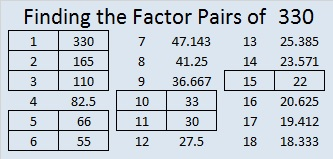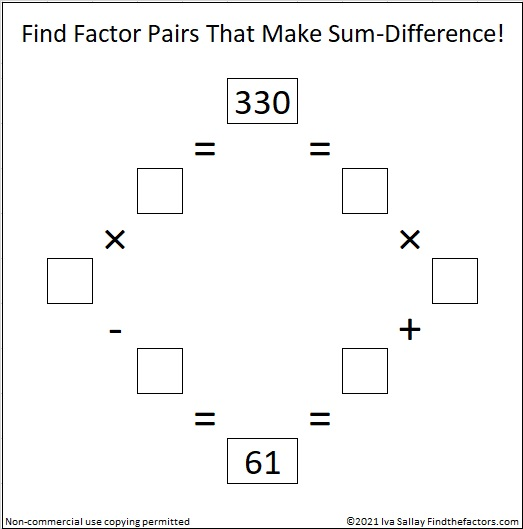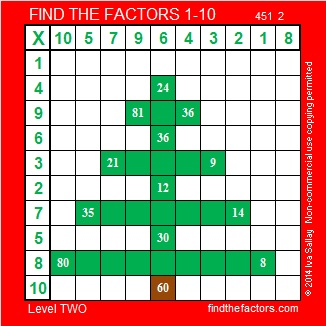# 330 Christmas Factor Trees

Contents

### Today’s Puzzle:

Can you find the factors and complete this Christmas tree multiplication table?Print the puzzles or type the factors on this excel file:  10 Factors 2014-12-22

### Factor Trees for 330:

Within these seven factor trees for 330 there are also factor trees for 6, 10, 15, 22, 30, 33, 55, 66, 110, and 165, the tops of which are all in brown. The prime factors of 330 are all in red.### Factors of 330:

• 330 is a composite number.
• Prime factorization: 330 = 2 x 3 x 5 x 11
• The exponents in the prime factorization are 1, 1, 1, and 1. Adding one to each and multiplying we get (1 + 1)(1 + 1)(1 + 1)(1 + 1) = 2 x 2 x 2 x 2 = 16. Therefore 330 has exactly 16 factors.
• Factors of 330: 1, 2, 3, 5, 6, 10, 11, 15, 22, 30, 33, 55, 66, 110, 165, 330
• Factor pairs: 330 = 1 x 330, 2 x 165, 3 x 110, 5 x 66, 6 x 55, 10 x 33, 11 x 30, or 15 x 22
• 330 has no square factors that allow its square root to be simplified. √330 ≈ 18.166### Sum-Difference Puzzle:

330 has eight factor pairs. The numbers in one of those pairs add up to 61, and the numbers in another one subtract to 61. If you can identify those factors, then you can solve this puzzle!### Tree Puzzle Solution:This site uses Akismet to reduce spam. Learn how your comment data is processed.